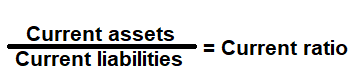# Current Ratio Calculator

Key in the Current Assets and Current Liabilities Values from your Balance Sheet to the respective fields given below and then click Calculate to get the desired result.

# Current Ratio Calculator - Glossary:

Current Ratio: Shows the proportion of current assets to current liabilities. In simple words, it measures a company's ability to pay the short-term liabilities with its current assets.

### Formula:### How to use this equation?

This is a balance sheet component; the values are commonly stated against current assets and current liabilities. To use this ratio, simply divide current assets with current liabilities.

Current Assets:
On the balance sheet, you will find the total value of current assets which consist of cash, cash equivalents, account receivables, merchandise inventory and marketable securities.

Current Liabilities:
On the balance sheet, you will find the total value of current liabilities which consist of accounts payable, tax payable (sales, payroll and other taxes) that are due in short-term.

Example:
Current ratio for a company with total current assets of \$200,000 and current liabilities of \$155,000 is 1.29:1. It means \$1.29 of current assets are available to cover each \$1 of its current liabilities.